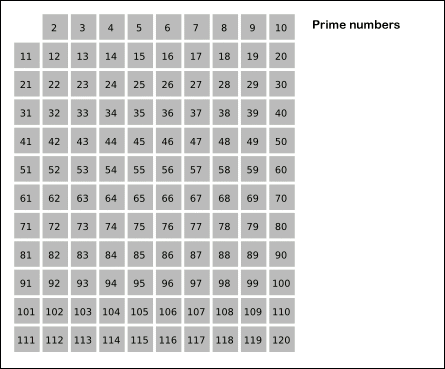### Sieve of Eratosthenes

In a previous article, we saw how to determine whether a number is a prime or not. Now, let's see how to find all primes smaller than or equal to $$n$$. We can run a loop from 1 to n and for each iteration check whether the loop counter variable is a prime or not. But that would be very inefficient since we would be repeating same calculations over and over again. In this situation it is best to use a method known as the Sieve of Eratosthenes. The Sieve of Eratosthenes will generate all the primes from 2 to n. It begins by assuming that all numbers are prime. It then takes the first prime number and removes all of its multiples. It then applies the same method to the next prime number. This is continued until all numbers have been processed.

This is a sieve. It's an utensil used for straining solids from liquids. Eratosthenes has devised such a sieve to strain prime numbers from first N numbers by draining out composite numbers.

Sift the Two's and Sift the Three's, The Sieve of Eratosthenes. When the multiples sublime, The numbers that remain are Prime.

## Demonstration of Sieve of Eratosthenes when $$n = 120$$,Source: Wiki

## Optimizing it,

Since all even numbers (except 2) are composite, we can stop checking even numbers at all. Instead, we need to operate with odd numbers only. First, it will allow us to half the needed memory. Second, it will reduce the number of operations performing by algorithm approximately in half.